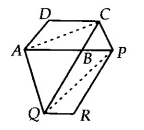Q

# The side AB of a parallelogram ABCD is produced to any point P. A line through A and parallel to CP meets CB produced at Q and then parallelogram PBQR is completed. Show that ar (ABCD) = ar (PBQR).

Q: 9         The side AB of a parallelogram ABCD is produced to any point P. A line through A and parallel to CP meets CB produced at Q and then parallelogram PBQR is completed (see Fig. ). Show that  .

[Hint: Join AC and PQ. Now compare   and  .]Views

Join the AC and PQ.It is given that ABCD is a ||gm and AC is a diagonal of ||gm
Therefore, ar(ABC) = ar(ADC) = 1/2 ar(||gm ABCD).............(i)

Also, ar(PQR) = ar(BPQ) = 1/2 ar(||gm PBQR).............(ii)

Since AQC and APQ are on the same base AQ and between same parallels AQ and CP.
ar(AQC) = ar (APQ)

Now, subtracting ABQ from both sides we get,

ar(AQC) - ar (ABQ) = ar (APQ) - ar (ABQ)
ar(ABC) = ar (BPQ)............(iii)

From eq(i), (ii) and (iii) we get

Hence proved.

Exams
Articles
Questions